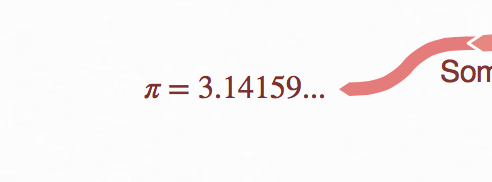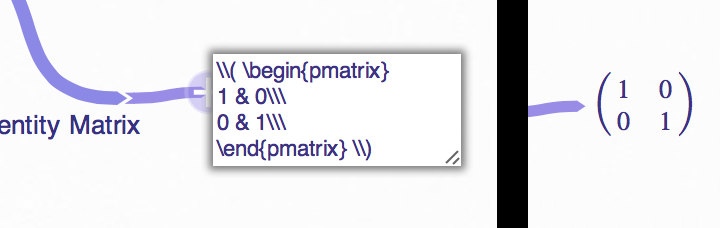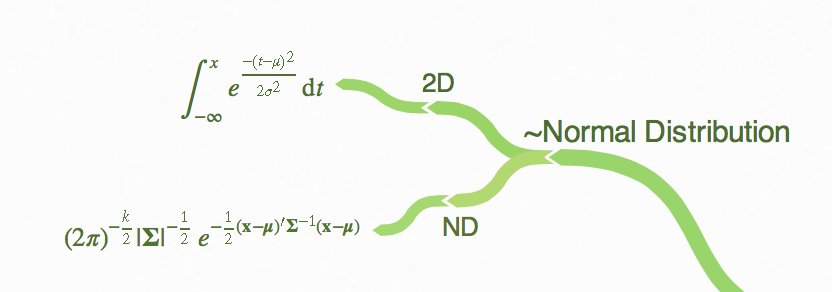# Add math formulae with LaTeX

Warning: this guide is recommended for advanced users and math students only! Here be LaTeX dragons.

Coggle supports the LaTeX math syntax (via MathJax) for adding math formulae and expressions to Coggle diagrams. This guide introduces some simple examples, we'd recommend the LaTeX Mathematics wikibook for a more advanced guide to LaTeX.

Note that Coggle only supports LaTeX math expressions, not text-mode commands.

### Simple Math Expressions

Here’s a really simple math expression to get started:Here the whole expression is actually entered into the text box asa simple LaTeX formula:

\$$\pi = 3.14159... \$$

That looks quite different to what’s displayed, but we’ll break it down. Firstly, the   \$$ and \$$ at the start and end mark the beginning and end of the block of math, that leaves us with:
\pi = 3.14159...


inside the math block. The next thing we get to is \pi, which is just the LaTeX way of writing the greek letter pi. You’ll notice that the backslash \ is used to start almost all special LaTeX expressions. Finally, we just have a plain equals sign, number, and some periods, which are all included in the displayed text with no special interpretation.

### Matrices

Here’s a more complex example of how to display a matrix, we’ll explain the syntax below:The syntax looks like this:

\$$\begin{pmatrix} 1 & 0 \\\ 0 & 1 \\\ \end{pmatrix} \$$


As before, all the text within \$$\$$ is interpreted as LaTeX. the \begin{matrix} ... \end{pmatrix} block defines the sort of matrix that will be displayed (here’s a list of others). & separates the columns of the matrix, and \\\ ends each row of the matrix. Note that there’s a small difference here to standard LaTeX: for technical reasons you need to use \\\ ( three backwards slashes) to end a line instead of the normal two.

### Something More Complex

Finally, let’s look at something more complex. Here’s something that’s important to lots of science and maths students because of it’s so widely applicable in statistics: the Normal Distribution.Pretty complex! Here’s the text to produce the 2D normal distribution:
\$\int_{-\infty}^{x} e^{\frac{-(t-\mu)^2}{2 \sigma^2}} \mathrm{d}t \$

And the text to produce the N-Dimensional normal distribution:
\$(2\pi)^{-\frac{k}{2}} |\boldsymbol\Sigma|^{-\frac{1}{2}} e^{ -\frac{1}{2}(\mathbf{x}-\boldsymbol\mu)'\boldsymbol\Sigma^{-1}(\mathbf{x}-\boldsymbol\mu) } \$


Immediately you’ll notice that we’ve used \$ and \$ to start the math block, instead of \$$ and \$$ – this means the LaTeX is interpreted as if it’s on its own line, rather than sharing a line with other text. (Try adding text outside the maths blocks to see the effect of this). We’ll leave a full explanation of the syntax to the reader, (you’ll find the LaTeX/Mathematics wikibook very helpful!),  but the best way to get started is just to copy and paste one of the expressions into a new Coggle of your own, edit the text and see what happens!

For the really interested, a full list of the supported LaTeX commands is available at mathjax.org, and you can see one of the Coggle team’s math demo Coggles here.